# Find Earliest or Latest Date Based on Criteria – Excel & Google Sheets

This tutorial will demonstrate how to use the MAXIFS and MINIFS Functions to identify the earliest and last dates that meet certain conditions in Excel and Google Sheets.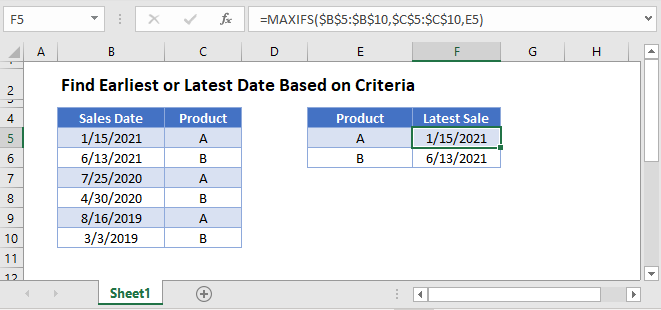## Find Latest Date with MAXIFS Function

First, we will show how to use the MAXIFS Function to identify the last relevant date from a cell range.

The MAXIFS Function outputs the largest value in a range that meets a specified criteria.

This example will show the latest Sales Date for each type of Product:

``=MAXIFS(B3:B8,C3:C8,E3)``The MAXIFS Function follows the same syntax style as the SUMIFS and AVERAGEIFS Functions..

To find the latest Sales Date for each Product that is before the date 12/31/2020, we can add a second criteria by using the DATE Function to define a date criteria:

``=MAXIFS(B3:B8,C3:C8,E3,B3:B8,"<"&DATE(2020,12,31))``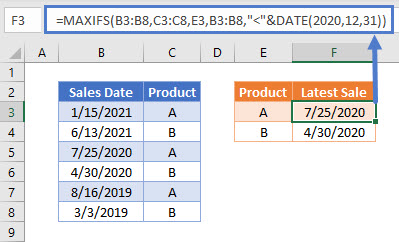## Finding Earliest Dates with MINIFS Function

In a similar way to the example above, we can use the MINIFS Function to identify the earliest relevant date from a cell range.

The MINIFS Function outputs the smallest value in a range that meets a specified criteria.

This example will show the earliest Sales Date for each type of Product:

``=MINIFS(B3:B8,C3:C8,E3)``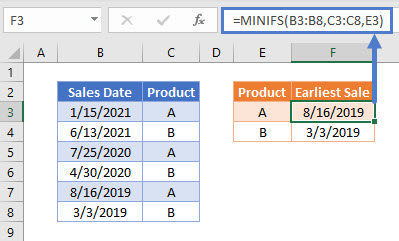## Locking Cell References

To make our formulas easier to read, we’ve shown the formulas without locked cell references:

``=MINIFS(B3:B8,C3:C8,E3)``

But these formulas will not work properly when copy and pasted elsewhere in your file. Instead, you should use locked cell references like this:

``=MINIFS(\$B\$3:\$B\$8,\$C\$3:\$C\$8,E3)``

## Alternative Method Using MAX and IF Functions

The MAXIFS and MINIFS Functions were added to Excel from 2007 onwards. Before this new release, you would need to use the MAX (or MIN) and IF Functions to produce the same result.

To show how this can be done, we can replicate the example to show the latest Sales Date for each type of Product using the MAX and IF Functions in an array formula:

``{=MAX(IF(C3:C8=E3,B3:B8))}``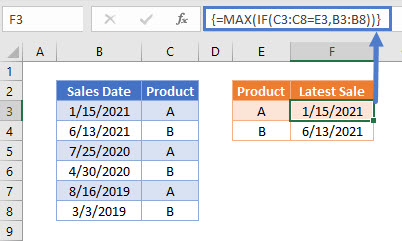As this example uses an array formula, it needs to be entered by pressing CTRL+SHIFT+ENTER. Doing this automatically shows { } array brackets around the formula. These do not need to be typed manually.

## Find Earliest or Latest Date Based on Criteria in Google Sheets

These formulas work exactly the same in Google Sheets as in Excel.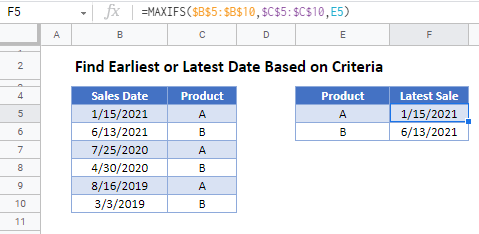### Excel Practice Worksheet

Practice Excel functions and formulas with our 100% free practice worksheets!